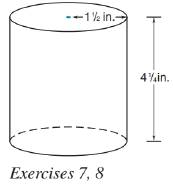Chapter 9.3, Problem 8E### Elementary Geometry for College St...

6th Edition
Daniel C. Alexander + 1 other
ISBN: 9781285195698

#### Solutions

Chapter
Section### Elementary Geometry for College St...

6th Edition
Daniel C. Alexander + 1 other
ISBN: 9781285195698
Textbook Problem
3 views

# What is the volume of the tin can? If it contains 16 oz of green beans, what is the volume of the can used for 20 oz green beans? Assume a proportionality between weight and volume.To determine

To calculate:

The volume of the tin can.

Explanation

Given:

A tin can shown below has the indicated dimensions and it can contain 16 oz of green beans.

Another tin can contains 20 oz of green beans.

Formula used:

The volume of the right circular cylinder is V=πr2h.

Calculation:

From the figure we see that, r=32in and h=174in.

Substitute the known values in the formula,

V=πr2h=π(32)2(174)=π(15316)=9.5625πV30

### Still sussing out bartleby?

Check out a sample textbook solution.

See a sample solution

#### The Solution to Your Study Problems

Bartleby provides explanations to thousands of textbook problems written by our experts, many with advanced degrees!

Get Started

#### Find more solutions based on key concepts